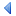UpTOC Index << 3 Using Euphoria < 4.4 Branching Statements Up: 4 Language Reference 4.6 Flow control statements > 5 Formal Syntax >>

## 4.5 Loop statements

An iterative code block repeats its own execution zero, one or more times. There are several ways to specify for how long the process should go on, and how to stop or otherwise alter it. An iterative block may be informally called a loop, and each execution of code in a loop is called an iteration of the loop.

Euphoria has three flavors of loops. They all may harbor a Header Labels, in order to make exiting or resuming them more flexible.

### 4.5.1 while statement

A while statement tests a condition to see if it is non-zero (true), and if so, a body of statements is executed. The condition is re-tested after when the statements are run, and if still true the statements are run again, and so on.

Syntax Format:

while expr [with entry] [label "name" ] do
statements
[entry]
statements
end while

Example 1

```while x > 0 do
a = a * 2
x = x - 1
end while
```

Example 2

```while sequence(Line) with entry do
proc(Line)
entry
Line = gets(handle)
end while
```

Example 3

```while true label "main" do
res = funcA()
if res > 5 then
if funcB() > some_value then
continue "main" -- go to start of loop
end if
procC()
end if
procD(res)
for i = 1 to res do
if i > some_value then
exit "main" -- exit the "main" loop, not just this 'for' loop.
end if
procF(i,res)
end if

res = funcE(res, some_value)
end while
```

### 4.5.2 loop until statement

A loop statement tests a condition to see if it is non-zero (true), and until it is true a loop is executed.

Syntax Format:

loop [with entry] [label "name" ] do
statements
until expr
end loop

```loop do
a = a * 2
x = x - 1
until x<=0
end loop
```
```loop with entry do
a = a * 2
entry
x = x - 1
until x<=0
end loop
```
```loop label "GONEXT" do
a = a * 2
y += 1
if y = 7 then continue "GONEXT" end if
x = x - 1
until x<=0
end loop
```

A while statement differs from a loop statement because the body of a loop is executed at least once, since testing takes place after the body completes. However in a while statement, the test is taken before the body is executed.

### 4.5.3 for statement

Syntax Format:

for loopvar = startexpr to endexpr [by delta] do
statements
end for

A for statement sets up a special loop that has its own loop variable. The loop variable starts with the specified initial value and increments or decrements it to the specified final value. The for statement is used when you need to repeat a set of statements a specific number of times.
Example:

```-- Display the numbers 1 to 6 on the screen.
puts(1, "1\n")
puts(1, "2\n")
puts(1, "3\n")
puts(1, "4\n")
puts(1, "5\n")
puts(1, "6\n")
```

This block of code simply starts at the first line and runs each in turn. But it could be written more simply and flexibly by using a for statement.

```for i = 1 to 6 do
printf(1, "%d\n", i)
end for
```

Now it's just three lines of code rather than six. More importantly, if we needed to change the program to print the numbers from 1 to 100, we only have to change one line rather than add 94 new lines.

```for i = 1 to 100 do -- One line change.
printf(1, "%d\n", i)
end for
```

Or using another way ...

```for i = 1 to 10 do
? i   -- ? is a short form for print()
end for

-- fractional numbers allowed too
for i = 10.0 to 20.5 by 0.3 do
for j = 20 to 10 by -2 do    -- counting down
? {i, j}
end for
end for
```

However, adding together floating point numbers that are not the ratio of an integer by a power of 2 -- 0.3 is not such a ratio--leads to some "fuzz" in the value of the index. In some cases, you might get unexpected results because of this fuzz, which arises from a common hardware limitation. For instance, floor(10*0.1) is 1 as expected, but floor(0.1+0.1+0.1+0.1+0.1+0.1+0.1+0.1+0.1+0.1) is 0.

The loop variable is declared automatically and exists until the end of the loop. Outside of the loop the variable has no value and is not even declared. If you need its final value, copy it into another variable before leaving the loop. The compiler will not allow any assignments to a loop variable. The initial value, loop limit and increment must all be atoms. If no increment is specified then +1 is assumed. The limit and increment values are established only on entering the loop, and are not affected by anything that happens during the execution of the loop.UpTOC Index << 3 Using Euphoria < 4.4 Branching Statements Up: 4 Language Reference 4.6 Flow control statements > 5 Formal Syntax >>